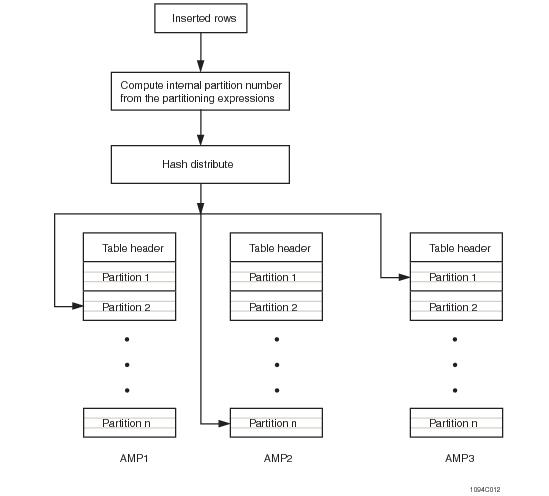# 15.00 - Row-Partitioned Row Allocation - Teradata Database

prodname
vrm_release
15.00
category
User Guide
featnum
B035-1094-015K

### Row‑Partitioned Row Allocation

If the primary index for your table is row partitioned, the following events occur when a row is processed for storage.

1 The row is hashed to its AMP (see “Hash-Based Table Partitioning to AMPs” on page 234) by the hash values of its primary index column set.

2 Its internal partition number is calculated by the AMP using software stored in the table header that computes the internal partition number from the row partitioning expressions defined for the table.

3 The file system inserts the row in logical order by internal partition number and hash value/uniqueness value.

If there are n row partitioning expressions, the rows are effectively grouped as follows:

a The value of the first row partitioning expression

b The value of the second row partitioning expression

c The value of the nth row partitioning expression

These row partitioning expression values are combined into a single combined partition that is mapped to an internal partition number that preserves their sequential order.

The final ordering is by the hash value of the primary index columns and its associated uniqueness value (see “Uniqueness Value” on page 227).

The following diagram provides a high-level picture of the process for a PPI table at the point where the rows have arrived at their assigned AMPs.